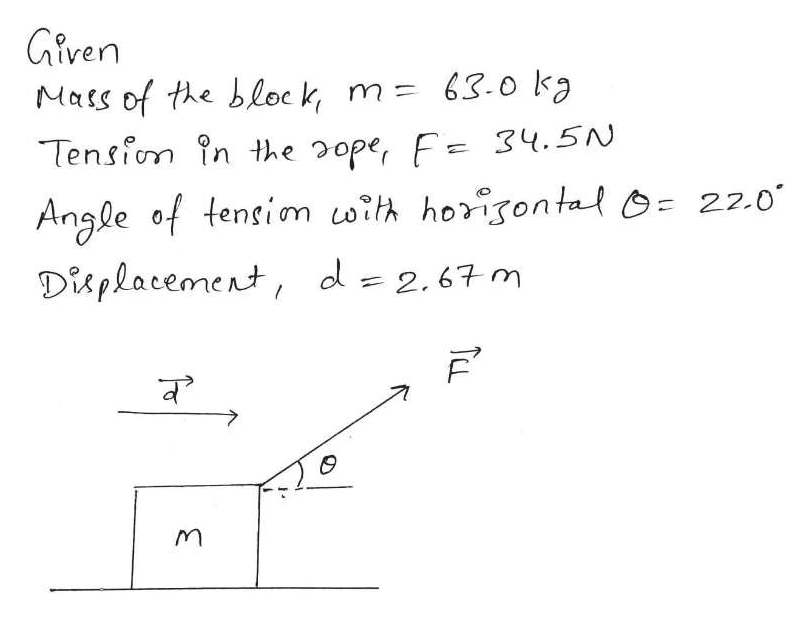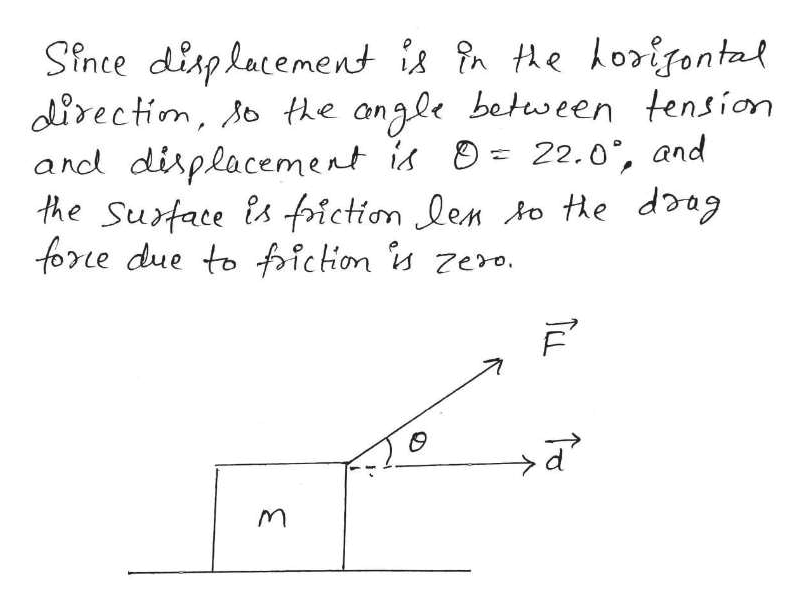# A 63.0-kg box is pulled by a rope making an angle of θ = 22.0° with the horizontal. The tension in the rope is 34.5 N. (Assume the surface the box slides across is frictionless.)If the box starts from rest, determine the time required to move the box a horizontal distance of 2.67 m.

Question

A 63.0-kg box is pulled by a rope making an angle of

θ = 22.0°

with the horizontal. The tension in the rope is

34.5 N.

(Assume the surface the box slides across is frictionless.)

If the box starts from rest, determine the time required to move the box a horizontal distance of 2.67 m.

check_circleExpert Solution
Step 1help_outlineImage TranscriptioncloseGiven 63.0 kg Mats of the block m Tensfon in the 2ope, F 34.5N 22.0 Angle of tensim oith horigon tal Displacement, d 2,67m व TCL fullscreen
Step 2help_outlineImage TranscriptioncloseSince disphacement is in the korifontal dlirectim, o the angle between tension and displacement is the Sutace is friction len to e doag fore due toichon zero. 22.0, and TCL fullscreen

### Want to see the full answer?

See Solution

#### Want to see this answer and more?

Solutions are written by subject experts who are available 24/7. Questions are typically answered within 1 hour*

See Solution
*Response times may vary by subject and question
Tagged in

### Work,Power and Energy